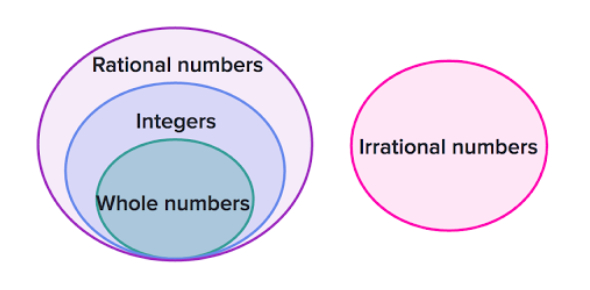# Integer Test Quiz: Math!

10 Questions | Total Attempts: 122Settings.

• 1.
If the temperature in Fairbanks is -9°C, and the temperature in Thule is 5° warmer.  What is the temperature in Thule?
• A.

4

• B.

14

• C.

-14

• D.

-4

• 2.
Which number sentence represents the numberline below?
• A.

(-3) + 2 = -1

• B.

2 + (-3) = 0

• C.

(-2) + (-1) = -3

• D.

3 + (-2) = 1

• 3.
If it is 5º outside and the temperature will drop 17º in the next six hours, how cold will it get?
• A.

12

• B.

-12

• C.

22

• D.

-17

• 4.
A scuba diver swam 96 feet beneath the surface of the lake. He then climbs up 49 feet. What is his depth now? [Blank]
• 5.
An elevator is on the twentieth floor. It goes down 11 floors and then up 5 floors. What floor is the elevator on now? [Blank]
• 6.
At midnight, the temperature was 30° F. By 6:00 a.m., it had dropped 5° and by noon, it had increased by 11°. What was the temperature at noon? [Blank]
• 7.
Use the model below to answer the question.
• A.

24

• B.

2

• C.

-2

• D.

-24

• 8.
Which equation is explained by the following model?
• A.

15 ÷ 3 = 5

• B.

3 ÷ 15 = 5

• C.

15 ÷ 5 = 3

• D.

3 x 5 = 15

• 9.
Which number sentence is explained by the model below?
• A.

6 x (-2) = 12

• B.

2 x (-6) = - 12

• C.

12 x 2 = 6

• D.

(-6) x (-2) = -12

• 10.
If the temperature on the thermometer rises by 45 degrees, the temperature will be [Blank]
Related TopicsBack to top